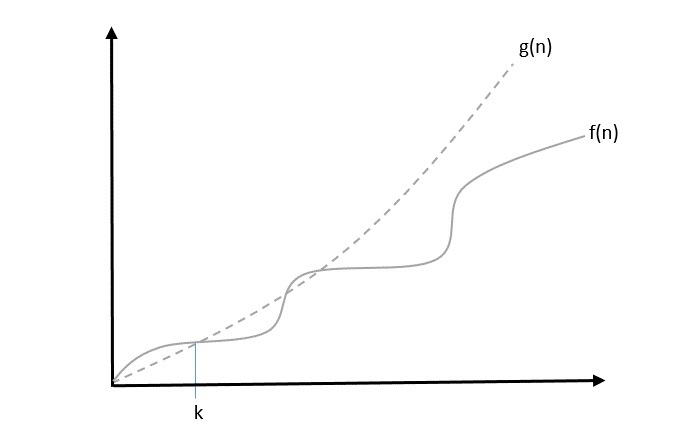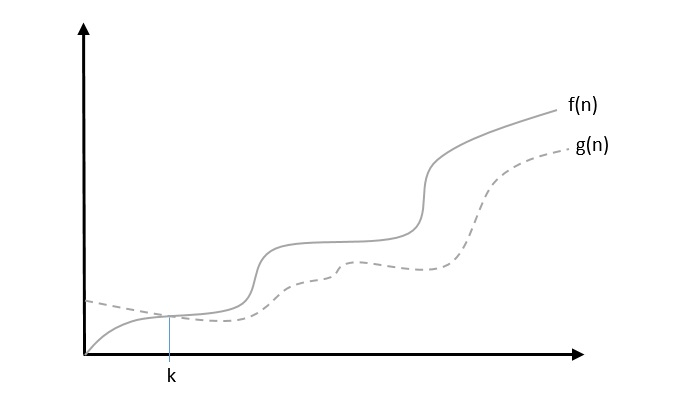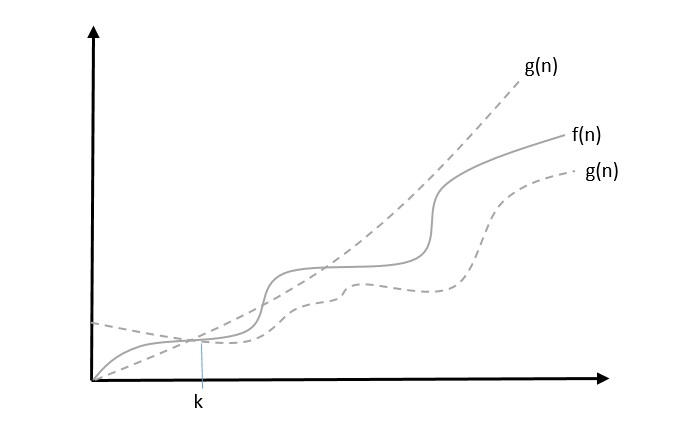# Data Structures - Asymptotic Analysis

Asymptotic analysis of an algorithm refers to defining the mathematical foundation/framing of its run-time performance. Using asymptotic analysis, we can very well conclude the best case, average case, and worst case scenario of an algorithm.

Asymptotic analysis is input bound i.e., if there's no input to the algorithm, it is concluded to work in a constant time. Other than the "input" all other factors are considered constant.

Asymptotic analysis refers to computing the running time of any operation in mathematical units of computation. For example, the running time of one operation is computed as f(n) and may be for another operation it is computed as g(n2). This means the first operation running time will increase linearly with the increase in n and the running time of the second operation will increase exponentially when n increases. Similarly, the running time of both operations will be nearly the same if n is significantly small.

Usually, the time required by an algorithm falls under three types −

• Best Case − Minimum time required for program execution.

• Average Case − Average time required for program execution.

• Worst Case − Maximum time required for program execution.

## Asymptotic Notations

Following are the commonly used asymptotic notations to calculate the running time complexity of an algorithm.

• Ο − Big Oh Notation

• Ω − Big Omega Notation

• Θ − Theta Notation

• o − Little Oh Notation

• ω − Little Omega Notation

### Big Oh Notation, Ο

The notation Ο(n) is the formal way to express the upper bound of an algorithm's running time. It measures the worst case time complexity or the longest amount of time an algorithm can possibly take to complete.For example, for a function f(n)

Ο(f(n)) = { g(n) : there exists c > 0 and n0 such that g(n) ≤ c.f(n) for all n > n0. }


### Example

Let us consider a given function, f(n) = 4.n3+10.n2+5.n+1.

Considering g(n) = n3

f(n) ≥ 5.g(n) for all the values of n > 2.

Hence, the complexity of f(n) can be represented as O (g (n) ) ,i.e. O (n3).

### Big Omega Notation, Ω

The notation Ω(n) is the formal way to express the lower bound of an algorithm's running time. It measures the best case time complexity or the best amount of time an algorithm can possibly take to complete.For example, for a function f(n)

Ω(f(n)) ≥ { g(n) : there exists c > 0 and n0 such that g(n) ≤ c.f(n) for all n > n0. }


### Example

Let us consider a given function, f(n) = 4.n3+10.n2+5.n+1

Considering g(n) = n3 , f(n) ≥ 4.g(n) for all the values of n > 0.

Hence, the complexity of f(n) can be represented as Ω (g (n) ) ,i.e. Ω (n3).

### Theta Notation, θ

The notation θ(n) is the formal way to express both the lower bound and the upper bound of an algorithm's running time. Some may confuse the theta notation as the average case time complexity; while big theta notation could be almost accurately used to describe the average case, other notations could be used as well. It is represented as follows −θ(f(n)) = { g(n) if and only if g(n) = Ο(f(n)) and g(n) = Ω(f(n)) for all n > n0. }


### Example

Let us consider a given function, f(n) = 4.n3+10.n2+5.n+1

Considering g(n) = n3 , 4.g(n)≤ f(n)≤ 5.g(n) for all the values of n.

Hence, the complexity of f(n) can be represented as Ɵ (g (n) ) ,i.e. Ɵ (n3).

### Little Oh (o) and Little Omega (ω) Notations

The Little Oh and Little Omega notations also represent the best and worst case complexities but they are not asymptotically tight in contrast to the Big Oh and Big Omega Notations. Therefore, the most commonly used notations to represent time complexities are Big Oh and Big Omega Notations only.

## Common Asymptotic Notations

Following is a list of some common asymptotic notations −

 constant − O(1) logarithmic − O(log n) linear − O(n) n log n − O(n log n) quadratic − O(n2) cubic − O(n3) polynomial − nO(1) exponential − 2O(n)